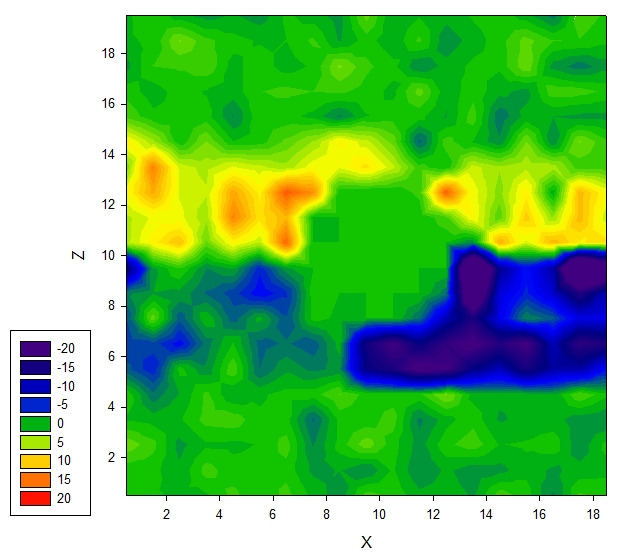# About per-atom stress

Hi, all,

I’m trying to use the per-atom stress output to generate local pressure map for my heterogeneous system (water-oil-solid particle). However, I’m getting unphysical data when solid is involved. I can’t figure out why.

I calculate the local pressure in the way similiar to this paper: Journal of applied physics 107,093517(2010) which is based on the original paper describing per-atom stress by Aidan Thompson(JCP 131, 154107 (2009)).

I run with DPD simulation where there is no long range electrostatic interaction so the per-atom stress should be calculated correctly

I first tested it with water-oil system where the two interfaces are perpendicular to the z axis. I plotted the gamma=(Pzz-0.5*(Pxx+Pyy)) in each bin. Everything makes sense and gamma show positive values only at the interfaces and zero value at the rest. The interfacial tension calculated through adding up the gamma in all the bins matches well with the interfacial tension calculated through the total pressure tensor

Then I tested a system with water and one solid nanoparticle (rigid body) at the center of the box. A restraining force is applied to the center of the particle. However, when I plot the gamma in each bins I get data shown in the contour below:figure above shows gamma values in xz plane that pass through the center of the solid spherical particle. The local pressure values near the solid nanoparticle is set to zero for clarity.

The gamma change from negative to positive when increasing z passing the center of the nanoparticle, which , in my opinion, is not physical at all. Firstly, there is only one phase, there is no interface of any kind other than the solid-liquid interface. Secondly, even if the change is due to the influence of the solid particle, should it be symmetric to the center of nanoparticle? (it is symmetric in xy plane) In DPD the interaction is short ranged (cutoff=1), I could not imaging what influenced the pressure where it is far away from the solid

I thought it could be because of the bugs in my code but I can’t figure out. Then I wrote another code that calculate the per-atom stress myself based on the pair force output (compute pair/local, the bond force was evaluated by myself). The part of the code that calculate the local pressure was kept exactly the same.

I tested it with water-oil system again got almost exactly the same result with direct per-atom stress output. Then I tested with the the above described system with one phase and a solid, everything looks normal (gamma got values only around the solid and other places got zero values) (figure not shown due to size limitation)

The only cause I can think of is either there is a bug in Lammps or I screw up some where (I tend to believe the later). But I really can’t figure it out. Can anyone help me with this? I don’t want to evaluate per-atom stress myself because it takes long time and large space for the pairforce data. It will be very convenient if I can use the per-atom stress output directly

I attached the input file of my system. I can provide the data file if anyone needs.

Sorry to bother

Thanks a lot

Heng

2011-09-22

input.LAMMPS (2.27 KB)

1 Like

You refer to Aidan’s paper and per-atom stress. The implementation
of per-atom stress in LAMMPS via the compute stress/arom command
is precisely what is in that paper. You can verify that it is “correct”
by summing the per-atom stress to get the total pressure, as described
on the compute doc page. I doubt there is a but with it.

Maybe Aidan wants to comment on your interpretation of the values.

SteveIf I understand correctly, you repeated the entire per-atom stress by a
different method, and got exactly the same result. Logically, that
indicates that LAMMPS' per-atom stress is working exactly as expected.
Thanks very much for doing that test. The source of your difficulties must
lie elsewhere. A fluid-fluid interface with a solid nanaoparticle is
actually a very complicated system. You should expect to be surprised.
Aidan

size limitation)
The only cause I can think of is either there is a bug in Lammps or I
screw
up some where (I tend to believe the later).

Good. Most people just stop with the former explanation. The fact that you
are willing to consider the latter gives me some hope that you will solve
the underlying problem.